## Introduction

Over 100 years ago Dimitri Mendeleev discovered that the atomic elements had periodic similarities, which when tabled allowed him to correctly guess the elements not yet discovered. As interest from fellow scientists grew the table soon filled and its emphasis towards discovery eventually died out, unfortunately its importance declined and had developed very little even though discoveries regarding the elements and their sub particles continued. Hence the desire to continue where others had left coupled with todays information produced this Progressive Periodic Table of the Elements (PPTE). Following are a few points which distinguishes this new table from the original.

## Points of Interest

1. The gaps between shell groups seem to show natural growth. When counting the horizontal lines of triangles, the Fibonacci progression stands out.
i.e. The addition of the last two numbers in the sequence is equal to the following number e.g.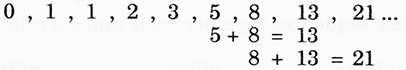2. A third line of axis links elements of similar sub shell number with different sub shell groups. i.e.3. In this table Hydrogen and Helium have dual ties with their sub shell groups s1, s2 and their similar outer shell groups p5, p6 , which has been a problem with tables of the past.
NB. Text books today use two tables to show this same information.

4. By counting the individual triangles from the grid, given that each triangle has a unit value of one (1), certain basic numerical progressions may be derived;

(a) The numbers on the left side of diagram 2 represents the total unit value within the region/area at each designated level(b) The total unites value prior to each shell group follows a similar sequence,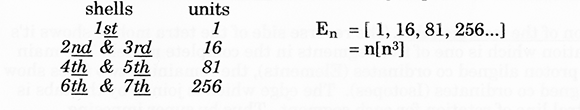(c) The maximum number of electrons that may exist per shell also follows this sequence of squares,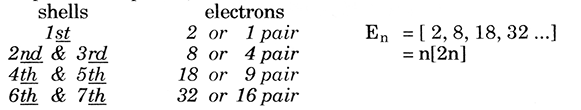(d) The number on the right side of diagram 2. represent the number of only upright triangle’s per level.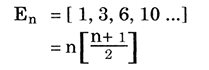(e) By dividing this sequence (separating every second number) two new sequences are revealed,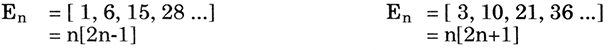(f) The number of orbitals/maximum number of electrons per sub shell follows a similar sequence,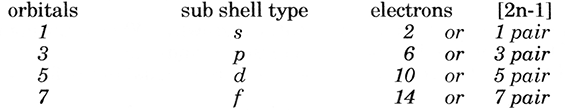(g) Finally, by placing these sequences together their mutual relationships become clear.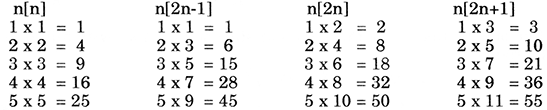5.The Inverse Square Law: explains the effect of two major characteristics consistent between electrons in which,
(i) It governs the distance of a stable orbit in proportion to its kinetic energy and
(ii) It derives the probability of locating the particle in a given area.
With this law in perspective, the PPTE on a mathematical basis complements the law remarkably. The simplicity of its structure along with its numerical advantage of ending each level with a square, allows it to function as an ideal frame for housing the elements and the laws that bind them. According to the data; number of elements, sub shells, electrons, even the distances between shells, all seem to be unambiguously accommodated by this table.

6.Transformation. From this point the PPTE advances to a more complex, three dimensional, representation adding two changes to it’s structure:
(i) The alignment of the elements into a continual line or spiral. This multi coloured spiral allows the observer to view the rotational action and continual link between shells.
(ii) The addition of the isotopic table. The reverse side of the tetra mobile shows it’s true representation which is one of five segments in the complete model. The main face shows the.proton aligned co ordinates (Elements), the remaining two faces show the neutron aligned co ordinates (Isotopes). The edge which is joined by the tabs is the axis or central line of rotation for each segment. Thus by super imposing multiple spirals around the axis shows the PPTE’s unique ability to align the elements with their isotopes as well as providing a continual link between the elements.

## Epilogue

Generally I believe this table allows students and readers alike to observe and learn the periodicity of the elements in a more aesthetically pleasing form, which takes confusion out of the growth pattern between shell pairs, and solves the problem of keeping the lanthanides and actinides in sequence with the rest of the table. It can easily be confined to an A4 sized page, thus deterring publishers from reconstructing it’s form.